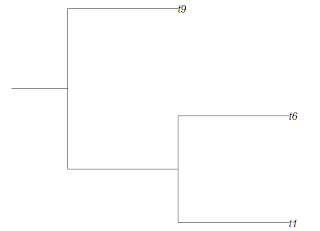## Wednesday, April 17, 2013

### New version of reroot

I just posted a new version of the function reroot, which I've moved to the source file utilities.R. The main different between reroot and the 'ape' function root is that reroot allows the user to root the tree along any internal or terminal edge, rather than just at nodes.

The previous version of this function had two problems: (1) it behaved incorrectly along edges arising from the root node of the tree; and (2) it depended on root(...,node=xx) which seems to act weird under some conditions & may have a bug. The new version of this function works totally differently. It still uses root - but with root(...,outgroup="XX") instead of using option node. This seems to fix the problems in the prior version. Instead, it uses the phytools functions splitTree to split the tree at the new root position, and then it reroots the basal subtree (i.e., the subtree rootward of the split point) at the tip, and then attaches the two subtrees together at this new root using the phytools function paste.tree.

Just for interest, here's a quick step-by-step demo of the process:

> require(phytools)
> # simulate tree
> tree<-rtree(n=10); tree\$edge.length<-rep(1,nrow(tree\$edge))
> plotTree(tree,node.numbers=T)> # let's re-root halfway along the branch ending in 18
> node.number<-18
> position=0.5*tree\$edge.length[which(tree\$edge[,2]==18)]
> # split the tree
> tt<-splitTree(tree,list(node=node.number,bp=position))
> plot(tt,no.margin=TRUE,root.edge=TRUE)Note that the top & bottom trees have a different scale - I show them for illustration of the split only.

> p<-tt[]; d<-tt[]
> # re-root the rootward subtree
> p<-root(p,outgroup="NA",resolve.root=T)
> # adjust branch lengths so that all the edge length
> # leading to "NA" is moved to the other side of the root
> bb<-which(p\$tip.label=="NA")
> ee<-p\$edge.length[which(p\$edge[,2]==bb)]
> p\$edge.length[which(p\$edge[,2]==bb)]<-0
> cc<-p\$edge[which(p\$edge[,2]==bb),1]
> dd<-setdiff(p\$edge[which(p\$edge[,1]==cc),2],bb)
> p\$edge.length[which(p\$edge[,2]==dd)]<- p\$edge.length[which(p\$edge[,2]==dd)]+ee
> plot(p,no.margin=TRUE,root.edge=TRUE)
> plot(d,no.margin=TRUE,root.edge=TRUE)> # re-attach
> tt<-paste.tree(p,d)
> plotTree(tt)That's it.

#### 1 comment:

1.Hi Liam,

I've got a quick question about rerooting a tip when the tip's parent is a polytomy. In this case, the reroot function produces a warning. I can't quite seem to figure out why, and I was wondering if you had any ideas. Here's a simple reproducible example:

> phy <- read.newick(text = "((A:1,B:1,C:1):1,(D:1,E:1):1);")
> rphy <- reroot(phy,node.number = 1,position = 0)
> rphy <- reorder(rphy,"postorder")
Warning messages:
1: In p\$edge[, 2] == dd :
longer object length is not a multiple of shorter object length
2: In p\$edge[, 2] == dd :
longer object length is not a multiple of shorter object length

When looking at the edges, it seems the polytomy isn't retained:

> rphy\$edge
[,1] [,2]
[1,] 8 4
[2,] 8 5
[3,] 7 8
[4,] 7 3
[5,] 6 7
[6,] 6 2
[7,] 6 1

Instead, I was thinking it might be:

[,1] [,2]
[1,] 8 4
[2,] 8 5
[3,] 7 8
[4,] 6 7
[5,] 6 3
[6,] 6 2
[7,] 6 1

but I'm not quite sure. Any thoughts?

Many thanks!

-Eric

Note: due to the very large amount of spam, all comments are now automatically submitted for moderation.